Select Page

# Maths 12 Science CBSE Probability Answers for MCQ in English

Maths 12 Science CBSE Probability Answers for MCQ in English to enable students to get Answers in a narrative video format for the specific question.

Expert Teacher provides Maths 12 Science CBSE Probability Answers for MCQ through Video Answers in English language. This video solution will be useful for students to understand how to write an answer in exam in order to score more marks. This teacher uses a narrative style for a question from Probability not only to explain the proper method of answering question, but deriving right answer too.

Please find the question below and view the Answer in a narrative video format.

Question:

## Similar Questions from CBSE, 12th Science, Maths, Probability

Question 1 :  A die is rolled. If E= (1, 3, 5), F(2, 3) and G= {2, 3, 4, 5}, find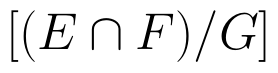(View Answer Video)

Question 2 : Consider the experiment of tossing a coin. If the coin shows head, toss is done again, but if it shows tail, then throw a die. Find the conditional probability of the events that ' the die shows a number greater than 4', given that ' there is atleast one tail'.    (View Answer Video)

Question 3 : If E and F be two evets such that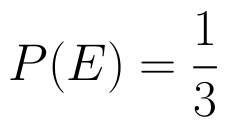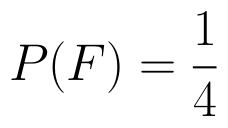, find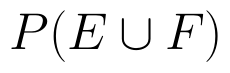if E and F are independent events. (View Answer Video)

Question 4 : An experiment succeeds thrice as often as it fails. Find the probability that in the next trials, there will be atleast 3 successes.    (View Answer Video)

Question 5 :  Probability of solving specific problem independently by A and B are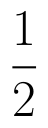and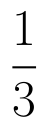respectively. If both try to solve the problem, independently, then find the probability that exactly one of them solves the problem.  (View Answer Video)

### Relations and Functions

Question 1 : Functions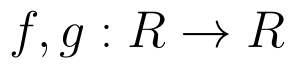are defined respectively, by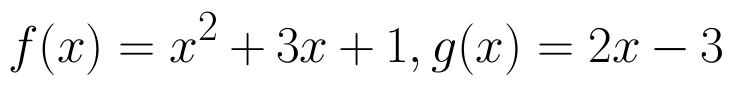, find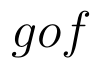(View Answer Video)

Question 2 :  A function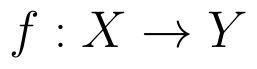is surjective if and only if , (View Answer Video)

Question 3 : If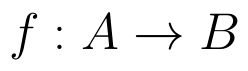and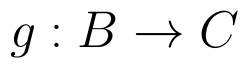are onto, then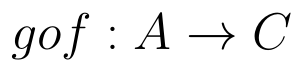is: (View Answer Video)

Question 4 : Consider a binary operation * on N defined as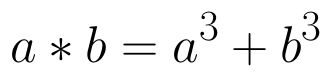. Choose the correct answer. (View Answer Video)

Question 5 :  If f is the greatest integer function and g is the modulus function . Write the value of g o f(-1/3) – f o g ( -1/3 ) . (View Answer Video)

### Matrices

Question 1 : Find the transpose of the matrix: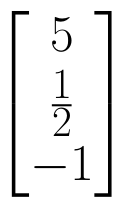. (View Answer Video)

Question 2 : Given,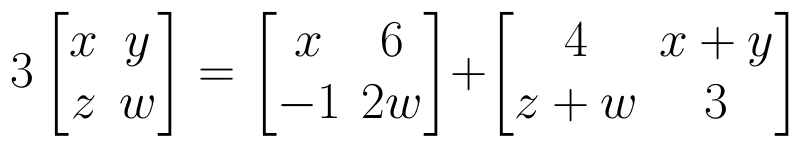, find the value of w. (View Answer Video)

Question 3 : Find the inverse of the matrix,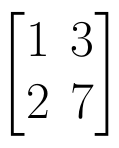. (View Answer Video)

Question 4 : If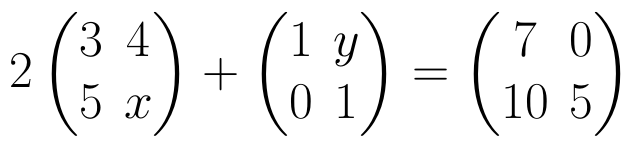, find (x-y). (View Answer Video)

Question 5 : Compute: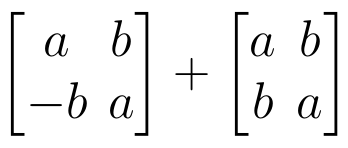. (View Answer Video)

### Three Dimensional Geometry

Question 1 : Find the distance of the point (-1, -5, -10) from the point of intersection of the line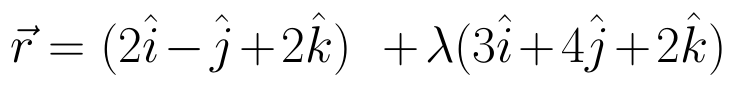and the plane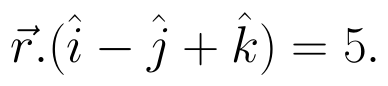(View Answer Video)

Question 2 : Find the distance between the point (7, 2, 4) and the plane determined by the points A(2, 5, -3), B(-2, -3, 5) and C(5, 3, -3). (View Answer Video)

Question 3 : If a line makes angles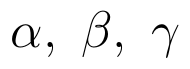with the positive direction of co-ordinate axes, then write the value of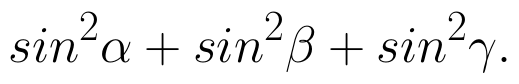(View Answer Video)

Question 4 : Find the distance of the point (-1, -5, -10) from the point of intersection of the line joining the points A(2, -1, 2) and B(5, 3, 4) with the plane x - y + z = 5. (View Answer Video)

Question 5 : Find the co-ordinates of the point, where the line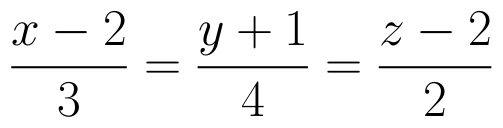intersects the plane x-y+z-5=0. Also find the angle between the line and the plane. (View Answer Video)